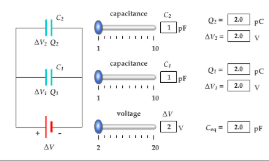# Problem: In the animation below, there are initially two capacitors and a battery connected in parallel. You can add up to two more parallel capacitors. Instructions: Use the blue sliders to adjust the battery voltage and individual capacitance values. Click the "add capacitor" box to add up to two more capacitors. Explore The capacitors in this animation are all connected in parallel. Circuit elements connected in parallel always have the same voltage. Since these capacitors are all connected in parallel with the battery, each has a voltage equal to the battery voltage. Capacitance is given by where Q is the charge on the capacitor's positive plate and V is the voltage across the capacitor. C= Q/V Capacitors connected in parallel can be replaced by an equivalent capacitor given by the following equation. C_eq = C_1 + C_2 + C_3 + ... Multiplying both sides of this equation by V results in the next equation. Q_eq = Q_1 + Q_2 + Q_3 + ... Thus, replacing the individual capacitors with C_eq causes the same amount of current to flow across the battery terminals. In each case below, three capacitors are connected in parallel across a 3 V battery. Part (a) uses capacitance values that can be set with the animation sliders, and you can use the animation to verify your calculation. Part (b) uses capacitance values outside the animation range. In each case, calculate C_eq and Q_eq. C_1 = 10 pF, C_2 = 6 pF, and C_3 = 2 pF. In each case below, three capacitors are connected in parallel across a 3 V battery. Part (a) uses capacitance values that can be set with the animation sliders, and you can use the animation to verify your calculation. Part (b) uses capacitance values outside the animation range. In each case, calculate C_eq and Q_eq. C_1 = 10 pF, C_2 = 6 pF, and C_3 = 2 pF. C_1 = 15 pF, C_2 = 11 pF, and C_3 = 11 pF.

###### FREE Expert Solution

The equivalent capacitance for capacitors in parallel is:

$\overline{){{\mathbf{C}}}_{\mathbf{e}\mathbf{q}}{\mathbf{=}}{{\mathbf{C}}}_{{\mathbf{1}}}{\mathbf{+}}{{\mathbf{C}}}_{{\mathbf{2}}}{\mathbf{+}}{\mathbf{.}}{\mathbf{.}}{\mathbf{.}}{\mathbf{+}}{{\mathbf{C}}}_{{\mathbf{n}}}}$

The charge stored in a capacitor:

$\overline{){\mathbf{Q}}{\mathbf{=}}{\mathbf{C}}{\mathbf{V}}}$

93% (67 ratings)###### Problem Details

In the animation below, there are initially two capacitors and a battery connected in parallel. You can add up to two more parallel capacitors.Instructions: Use the blue sliders to adjust the battery voltage and individual capacitance values. Click the "add capacitor" box to add up to two more capacitors. Explore The capacitors in this animation are all connected in parallel. Circuit elements connected in parallel always have the same voltage. Since these capacitors are all connected in parallel with the battery, each has a voltage equal to the battery voltage. Capacitance is given by where Q is the charge on the capacitor's positive plate and V is the voltage across the capacitor. C= Q/V Capacitors connected in parallel can be replaced by an equivalent capacitor given by the following equation. C_eq = C_1 + C_2 + C_3 + ... Multiplying both sides of this equation by V results in the next equation. Q_eq = Q_1 + Q_2 + Q_3 + ... Thus, replacing the individual capacitors with C_eq causes the same amount of current to flow across the battery terminals. In each case below, three capacitors are connected in parallel across a 3 V battery. Part (a) uses capacitance values that can be set with the animation sliders, and you can use the animation to verify your calculation. Part (b) uses capacitance values outside the animation range. In each case, calculate C_eq and Q_eq. C_1 = 10 pF, C_2 = 6 pF, and C_3 = 2 pF. In each case below, three capacitors are connected in parallel across a 3 V battery.

Part (a) uses capacitance values that can be set with the animation sliders, and you can use the animation to verify your calculation. Part (b) uses capacitance values outside the animation range. In each case, calculate C_eq and Q_eq. C_1 = 10 pF, C_2 = 6 pF, and C_3 = 2 pF. C_1 = 15 pF, C_2 = 11 pF, and C_3 = 11 pF.